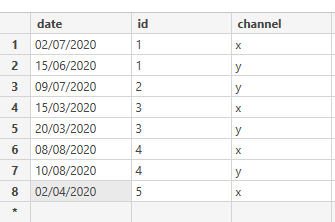cancel
Showing results for
Did you mean:Anonymous
Not applicable

## count with filter

Dear All,

I would like to count id if id date >= 01/07/2020 for both channel. The returned result will be 1 which is id 4. How can I write the measure column for this please help.

Thanks1 ACCEPTED SOLUTIONit's quite tricky. You should write a measure, not a column. The principle in DAX is "filter first, evaluate second". So you could try this (I haven't tested it).

``````=
CALCULATE (
SUMX ( values(tablename[id]), IF ( calculate(countrows ( tablename[id] )) > 1, 1 ) ),
FILTER ( tablename, tablename[date] >= DATE ( 2020, 7, 1 ) )
)``````

* Matt is a Microsoft MVP (Power BI) and author of the Power BI Book Supercharge Power BI.
6 REPLIES 6it's quite tricky. You should write a measure, not a column. The principle in DAX is "filter first, evaluate second". So you could try this (I haven't tested it).

``````=
CALCULATE (
SUMX ( values(tablename[id]), IF ( calculate(countrows ( tablename[id] )) > 1, 1 ) ),
FILTER ( tablename, tablename[date] >= DATE ( 2020, 7, 1 ) )
)``````

* Matt is a Microsoft MVP (Power BI) and author of the Power BI Book Supercharge Power BI.Anonymous
Not applicable

Actually I missed a point in my question that some id has only one channel also I need to check on. For examble below table ,if id has two channles both has to be greater than 01/07/2020 and if only one channel then it has to be greater than 01/07/2020. So for below table it should return 2 which ids 2 and 4. Please help. Thank you``````=
SUMX (
VALUES ( Table[id] ),
VAR countOfChannels =
CALCULATE ( DISTINCTCOUNT ( Table[channel] ) )
VAR countAfterDate =
CALCULATE ( COUNTROWS ( FILTER ( Table, [date] > DATE ( 2020, 7, 1 ) ) ) )
RETURN
IF ( countOfChannels = countAfterDate, 1 )
)``````

* Matt is a Microsoft MVP (Power BI) and author of the Power BI Book Supercharge Power BI.Anonymous
Not applicable

Actually I am getting an error  ThanksThere is nothing wrong with the syntax. What is the name of your table in the model?  Is it Table?  If not, you need to change "table" in this measure to match the name of your table.

* Matt is a Microsoft MVP (Power BI) and author of the Power BI Book Supercharge Power BI.Anonymous
Not applicable

Actually I did fix the formula but I got blank results. What is wrong with that can you tell me that please? Thank you

``````= SUMX (
VALUES('Table'[id]);
VAR countOfChannels
= CALCULATE(DISTINCTCOUNT ( 'Table'[channel] ) )
VAR countAfterDate
=CALCULATE ( COUNTROWS('Table');FILTER('Table';'Table'[date]> DATE(2020;7;1)))
RETURN
IF ( countOfChannels = countAfterDate;1)
)``````